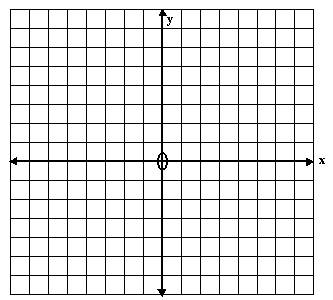# Plotting Straight Line Graphs using Gradient Method Tutorial

math tutorials > plotting straight line graphs using gradient method

## What is the gradient of a straight line graph?

The gradient of a line (symbol m) gives us information  about the steepness of a line and the direction in which it slopes.

## Example

In  y = ½ x + 1,   the gradient is ½.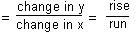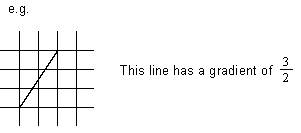## Note:

1. Vertically, a step up is positive, a step down is negative.  Horizontally, a step to the right is positive, to the left is negative.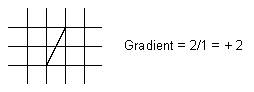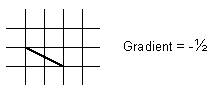2. A horizontal line has a gradient of 0.
3. A vertical line is infinitely steep.  The gradient is undefined.

## Drawing a graph form the equation y = mx + c.

2. Identify and plot the y-intercept (c).
3. Draw a line form the y-intercept with the correct gradient.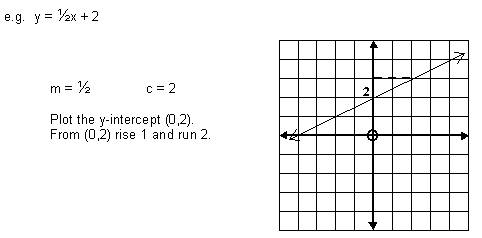## Graph problems for you to try

You may need to rearrange the equation into the form y = mx + c.

1. y = x + 2
2. y = -2x + 3
3. y = ½x - 4
4. x = y = 6
5. 2x + 5y = 10